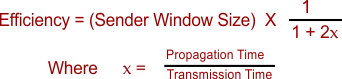Select Page

# Numerical of Flow Control Protocols

In Numerical of Flow Control Protocols, mostly need to calculate efficiency. Before to calculate efficiency, lets learn all basic factors in protocols

 Stop and Wait Go Back N Selective Repeat Only one frame can transmit at a time Multiple frame transmission at a time Multiple frame transmission at a time Sender window size =1 Sender window size = 2K-1 Sender window size = 2K-1 Receiver window size =1 Receiver window size =1 Receiver window size = 2K-1

Note: K is the number of bits to represent the window size)

To find the Efficiency of any protocol use the any of following formulaHelp Other’s By Sharing…